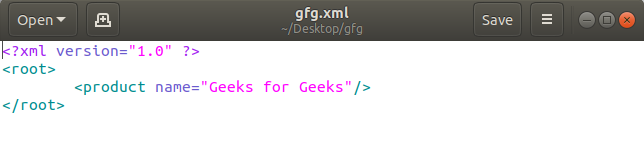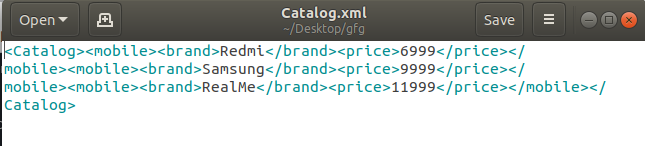# Create XML Documents using Python

• Difficulty Level : Expert
• Last Updated : 10 May, 2020

Extensible Markup Language(XML), is a markup language that you can use to create your own tags. It was created by the World Wide Web Consortium (W3C) to overcome the limitations of HTML, which is the basis for all Web pages. XML is based on SGML – Standard Generalized Markup Language. It is used for storing and transporting data. XML doesn’t depend on the platform and the programming language. You can write a program in any language on any platform to send, receive, or store data using XML. It defines a set of rules for encoding documents in a format that is both human-readable and machine-readable.

Data is stored in XML documents. XSL(eXtensible Stylesheet Language) documents describe how to change XML documents into other kinds of documents (such as HTML, TXT or even XML.) The process of transformation is called XSLT, or sometimes XSL transformations.

Example:

 ```<``message``>    ``<``from``> Robert    ``<``to``> Mario ```

## Creating XML Document using Python

1) Creating XML document using minidom
First, we import `minidom` for using `xml.dom`. Then we create the `root` element and append it to the XML. After that creating a child product of parent namely Geeks for Geeks. After creating a child product the filename is saved as ‘any name as per your choice.xml’. Do not forget to append .xml at the end of the filename you have given to the file. Minidom is a minimal implementation of the Document Object Model interface, with an API similar to that in other languages. It is intended to be simpler than the full DOM and also significantly smaller.

Example:

 `from` `xml.dom ``import` `minidom``import` `os `` ` ` ` `root ``=` `minidom.Document()`` ` `xml ``=` `root.createElement(``'root'``) ``root.appendChild(xml)`` ` `productChild ``=` `root.createElement(``'product'``)``productChild.setAttribute(``'name'``, ``'Geeks for Geeks'``)`` ` `xml.appendChild(productChild)`` ` `xml_str ``=` `root.toprettyxml(indent ``=``"\t"``) `` ` `save_path_file ``=` `"gfg.xml"`` ` `with ``open``(save_path_file, ``"w"``) as f:``    ``f.write(xml_str) `

Output:2) Creating XML document using ElementTree
Firstly we have to import `'xml.etree.ElementTree'` for creating a subtree. After that, we make root element, and that root element should be in an intended block otherwise the error will arise. After creating the root element, we can create a tree structure easily. Then the file will be stored as ‘name you want to give to that file.xml’. ElementTree is an important Python library that allows you to parse and navigate an XML document. Using ElementTree, we can break a document in a tree structure that is easy to work with.

Example:

 `import` `xml.etree.ElementTree as gfg `` ` ` ` `def` `GenerateXML(fileName) :``     ` `    ``root ``=` `gfg.Element(``"Catalog"``)``     ` `    ``m1 ``=` `gfg.Element(``"mobile"``)``    ``root.append (m1)``     ` `    ``b1 ``=` `gfg.SubElement(m1, ``"brand"``)``    ``b1.text ``=` `"Redmi"``    ``b2 ``=` `gfg.SubElement(m1, ``"price"``)``    ``b2.text ``=` `"6999"``     ` `    ``m2 ``=` `gfg.Element(``"mobile"``)``    ``root.append (m2)``     ` `    ``c1 ``=` `gfg.SubElement(m2, ``"brand"``)``    ``c1.text ``=` `"Samsung"``    ``c2 ``=` `gfg.SubElement(m2, ``"price"``)``    ``c2.text ``=` `"9999"``     ` `    ``m3 ``=` `gfg.Element(``"mobile"``)``    ``root.append (m3)``     ` `    ``d1 ``=` `gfg.SubElement(m3, ``"brand"``)``    ``d1.text ``=` `"RealMe"``    ``d2 ``=` `gfg.SubElement(m3, ``"price"``)``    ``d2.text ``=` `"11999"``     ` `    ``tree ``=` `gfg.ElementTree(root)``     ` `    ``with ``open` `(fileName, ``"wb"``) as files :``        ``tree.write(files)`` ` `# Driver Code``if` `__name__ ``=``=` `"__main__"``: ``    ``GenerateXML(``"Catalog.xml"``)`

Output:There are three helper functions that are useful for creating a hierarchy of Elementnodes. `Element()` function creates a standard node, `SubElement()` function attaches a new node to a parent node, and `Comment()` function creates a node that serializes using XML’s comment syntax.

The attribute values can be configured one at a time `withset()` (as with the root node), or all at once by passing a dictionary to the node factory(as with each group and podcast node).

My Personal Notes arrow_drop_up